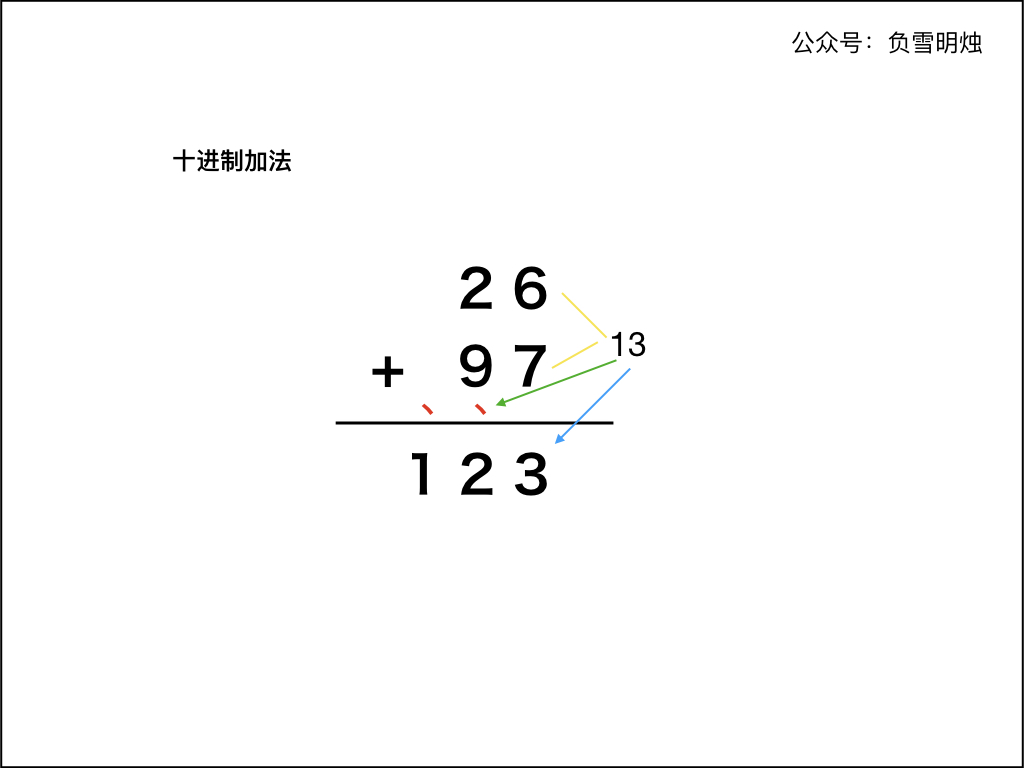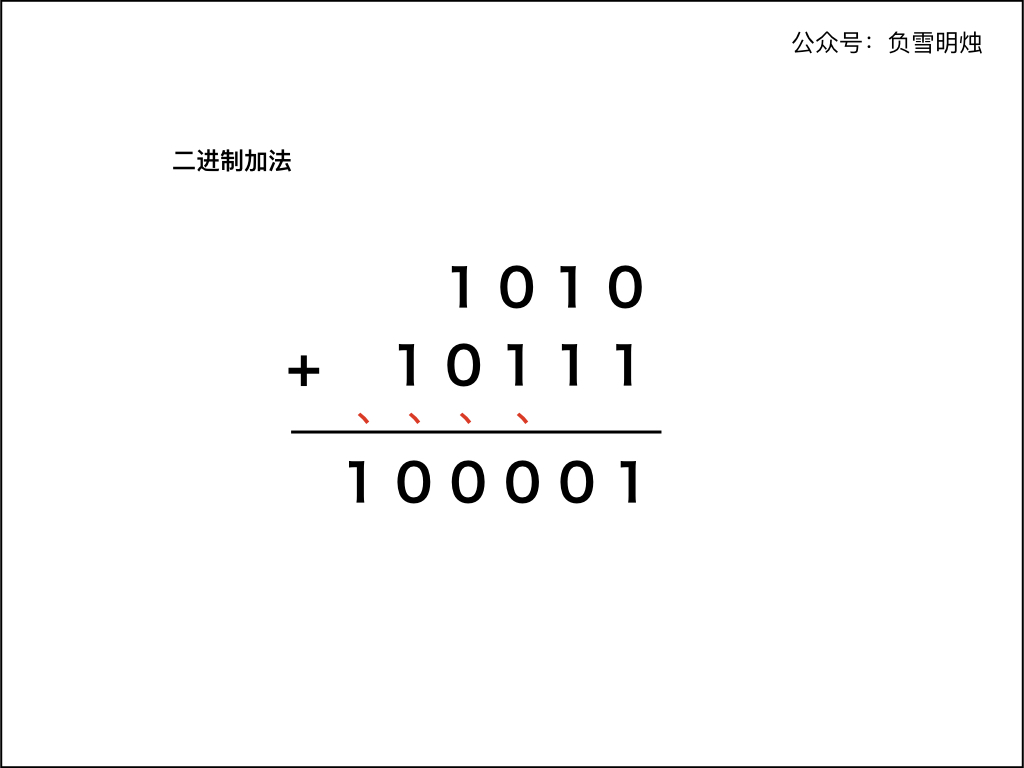• 作者： 负雪明烛
• id： fuxuemingzhu
• 公众号：负雪明烛
• 本文关键词：LeetCode，力扣，算法，算法题，二进制相加，字符串相加，整数加法，刷题群，Python, C++, Java

@TOC

# # 题目描述

Given two binary strings, return their sum (also a binary string).

The input strings are both non-empty and contains only characters 1 or 0.

Example 1:

Input: a = "11", b = "1"
Output: "100"


Example 2:

Input: a = "1010", b = "1011"
Output: "10101"


# # 解题方法

## # 解题方法：模拟法

### # 十进制加法1. 两个「加数」的右端对齐；
2. 从最右侧开始，依次计算对应的两位数字的和。如果和大于等于 10，则把和的个位数字计入结果，并向前面进位。
3. 依次向左计算对应位置两位数字的和，如果有进位需要加上进位。如果和大于等于 10，仍然把和的个位数字计入结果，并向前面进位。
4. 当两个「加数」的每个位置都计算完成，如果最后仍有进位，需要把进位数字保留到计算结果中。

### # 二进制加法

• 红点表示进位。## # 代码

### # 在代码中需要注意的有：

1. 本题给出的二进制数字是字符串形式，不可以转化成 int 型，因为可能溢出
2. 两个「加数」的字符串长度可能不同；
3. 在最后，如果进位 carry 不为 0，那么最后需要计算进位
4. 向结果字符串 res 拼接的顺序是向后拼接，返回时需要把 res 反转

### # 代码中的巧妙之处：

1. while (i >= 0 || j >= 0 || carry != 0)含义：
• 字符串 ab 只要有一个没遍历完，那么就继续遍历；
• 如果字符串 ab 都遍历完了，但是最后留下的进位 carry != 0，那么需要把进位也保留到结果中。
2. digit 的时候，如果字符串 ab 中有一个已经遍历完了（即 或者 ），则认为 ab 的对应位置是

Java 语言的代码如下，有详细的注释：

class Solution {
public String addBinary(String a, String b) {
StringBuilder res = new StringBuilder(); // 返回结果
int i = a.length() - 1; // 标记遍历到 a 的位置
int j = b.length() - 1; // 标记遍历到 b 的位置
int carry = 0; // 进位
while (i >= 0 || j >= 0 || carry != 0) { // a 没遍历完，或 b 没遍历完，或进位不为 0
int digitA = i >= 0 ? a.charAt(i) - '0' : 0; // 当前 a 的取值
int digitB = j >= 0 ? b.charAt(j) - '0' : 0; // 当前 b 的取值
int sum = digitA + digitB + carry; // 当前位置相加的结果
carry = sum >= 2 ? 1 : 0; // 是否有进位
sum = sum >= 2 ? sum - 2 : sum; // 去除进位后留下的数字
res.append(sum); // 把去除进位后留下的数字拼接到结果中
i --;  // 遍历到 a 的位置向左移动
j --;  // 遍历到 b 的位置向左移动
}
return res.reverse().toString(); // 把结果反转并返回
}
}


C++ 代码如下：

class Solution {
public:
string addBinary(string a, string b) {
string res;
int carry = 0;
int i = a.size() - 1;
int j = b.size() - 1;
while (i >= 0 || j >= 0 || carry != 0) {
int digitA = i >= 0 ? a[i] - '0' : 0;
int digitB = j >= 0 ? b[j] - '0' : 0;
int sum = digitA + digitB + carry;
carry = sum >= 2 ? 1 : 0;
sum = sum >= 2 ? sum - 2 : sum;
res += to_string(sum);
i --;
j --;
}
reverse(res.begin(), res.end());
return res;
}
};


Python 代码如下：

class Solution(object):
res = ""
carry = 0
i = len(a) - 1
j = len(b) - 1
while i >= 0 or j >= 0 or carry != 0:
digitA = int(a[i]) if i >= 0 else 0
digitB = int(b[j]) if j >= 0 else 0
sum = digitA + digitB + carry
carry = 1 if sum >= 2 else 0
sum = sum - 2 if sum >= 2 else sum
res += str(sum)
i -= 1
j -= 1
return res[::-1]


• 时间复杂度： 分别是字符串 ab 的长度；
• 空间复杂度：，只使用了常数的空间。

# # 总结

1. 加法」系列题目都不难，其实就是 「列竖式」模拟法
2. 需要注意的是 while循环结束条件，注意遍历两个「加数」不要越界，以及进位。

# # 日期

2017 年 8 月 17 日 2018 年 11 月 24 日 —— 周六快乐 2021 年 11 月 3 日 —— 把 Leetcode 上所有的加法题目都更新完了